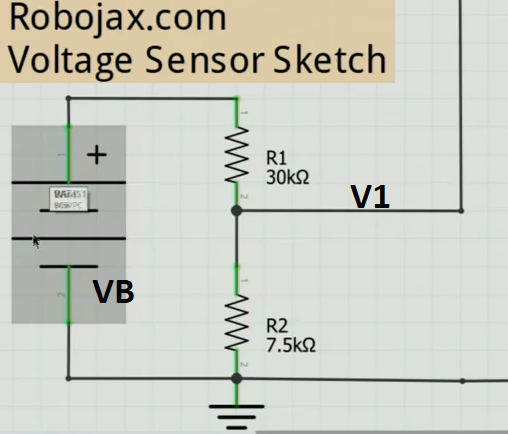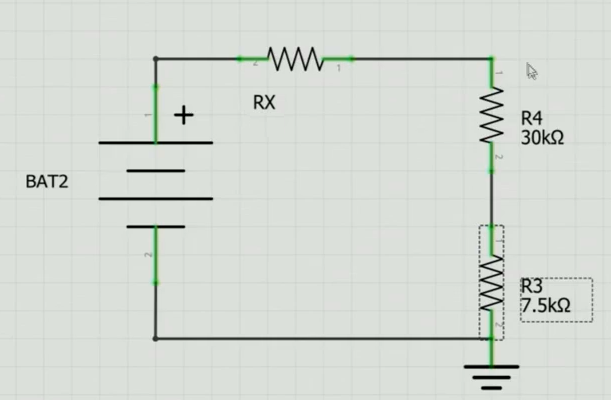# Voltage Sensor Standard Module for Arduino

• 5.00 AED
• Ex Tax:5.00 AED
• Product Code: Digital
• Availability: In Stock
This sensor is able to measure a voltage up to 25V when it's powered by 5V supply. The module also includes convenient screw terminals for easy and secure connection of a wire.This module is based on principle of resistive voltage divider design, can make the red terminal connector input voltag..
This sensor is able to measure a voltage up to 25V when it's powered by 5V supply. The module also includes convenient screw terminals for easy and secure connection of a wire.

This module is based on principle of resistive voltage divider design, can make the red terminal connector input voltage to 5 times smaller.Arduino analog input voltages up to 5 v,

Specification:

Voltage input range : DC0-25 V

Voltage detection range : DC0.02445 V-25 V

This video shows you how to Use Arduino Voltage Sensor. You will learn how to measure 25V DC but trick is shown so you can measure virtually any voltage Down below :

## This is the Arduino code and video for Voltage Sensor module

This video shows you how to use Voltage Sensor module and also how to use it to measure any voltage by showing you how to calculate the resistor.``````
/*
* This is the Arduino code for  Voltage Sensor
* you will measure voltage using this module which eliminate use extra resistors
in the video also you will learn how to measure any voltages.

* Watch the video instruction for Arduino Voltage sensor https://youtu.be/FaoKzi7tgME
*  *

* note is kept with the code.
* Disclaimer: this code is "AS IS" and for educational purpose only.
*
*/
/*
* Written by Ahmad Shamshiri for Robojax
* on Feb 07, 2018 at 20:05, Ajax, Ontario, Canada
*/
int offset =20;// set the correction offset value
void setup() {
// Robojax.com voltage sensor
Serial.begin(9600);
}

void loop() {
// Robojax.com voltage sensor
double voltage = map(volt,0,1023, 0, 2500) + offset;// map 0-1023 to 0-2500 and add correction offset

voltage /=100;// divide by 100 to get the decimal values
Serial.print("Voltage: ");
Serial.print(voltage);//print the voltge
Serial.println("V");

delay(500);

}
```  ```

How to make Arduino Voltage Sensor

Voltage divider is used to calculate the the voltage for arduino voltage sensor as shown in Fig. 1

In this figure we have got:

Battery voltage VB

Output voltage to that goes to Arduino V1

R1 is 30kΩ

R2 is 7.5kΩFigure 1. Voltage divider for Arduino voltage sensor

The maximum voltage we can calculate with this circuit is when V1 is 5V. From this we can find the current I in both R1 and R2

I=VR and therefore I=57500=0.000666=666µA

Because R1 and R2 are in series, the current is the same for both. So IR2 is also  666µA. We can find the voltage across R1 as

VR1=666µ x 30000=20V.

Now we know the maximum voltage at V1 can be

VR1+VR2=5V+20V=25V.

## Calculating Larger Voltage Values

We can add another resistor in series RX with this  circuit as shown in Figure 2.Figure 2. Series Resistor RX in circuit.

To find the value of RX, to measure know BAT2 Voltage, we have:

RX=BAT2-25666uA

Now We have got the formula for RX, if for example BAT2 = 120V

Then

RX=120-25666uA=95666ua=142.5kΩ

So using 142kΩ resistor as RX, we can measure up to maximum of 120V.

Tags: module; power; sensors; voltage

There are no reviews for this product.